Learning Library

# Math Mania! Our Best Second Grade Worksheets

Sharpen second grade math skills with this collection of worksheets, featuring addition, time, place value, fractions and more.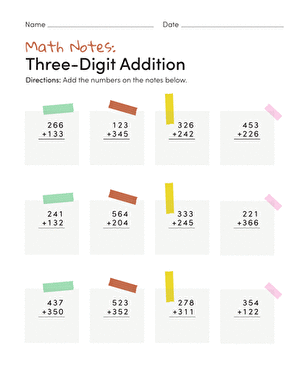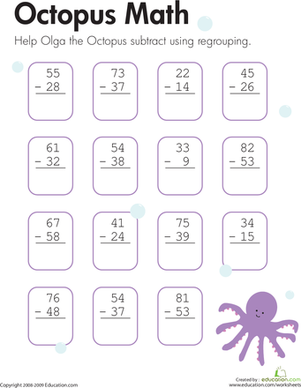### Subtraction with Regrouping: Octopus Math

Kids help Olga the Octopus on this second grade math worksheet by solving subtraction problems with two-digit numbers using regrouping.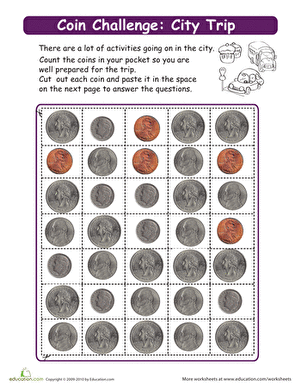### Make Change: City Trip!

In this worksheet, students practice adding, subtracting, and working with coins during a trip to the city.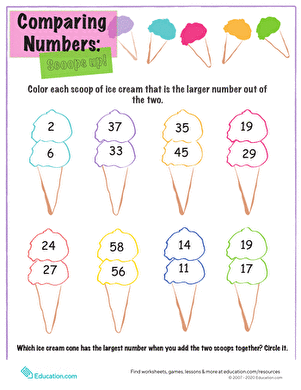### Comparing Numbers: Scoop's Up

On this worksheet, kids compare the numbers written on each scoop of ice cream and color in the scoop with the biggest number.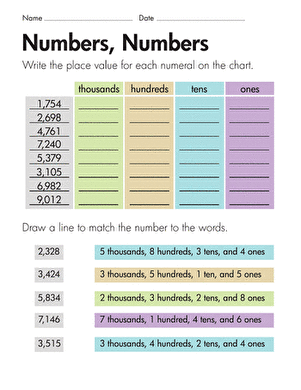### Place Value: Numbers, Numbers

For each four-digit number on this second grade math worksheet, kids determine the place value of each digit.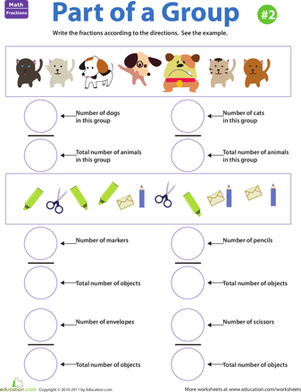### Fraction Practice: Part of a Group #2

Kids usually think of fractions as a part of a whole, but they can also describe a part of a group. This worksheet helps kids grasp this tricky concept.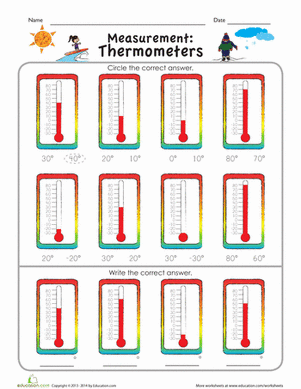### Measurement Mania: Thermometers

Turn up the heat with this measurement worksheet that helps kids learn how to read a thermometer.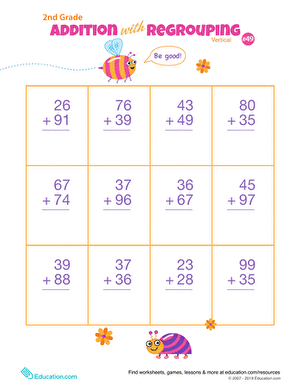### Double Digits! Practice Vertical Addition with Carrying 49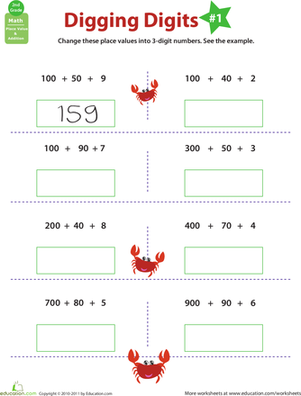### Digging Digits: Practice Place Value #1

Start your second grader out with some place value practice with this colorful worksheet that explores the ones, tens, and hundreds digits.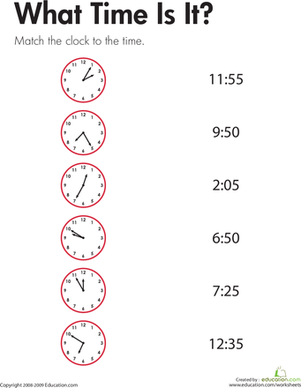### Telling Time: What Time Is It?

For this second grade math worksheet, kids look at each analog clock, determine the time, and draw a line to connect the clock with the digital time.

Create new collection

0

### New Collection>

0 items

What could we do to improve Education.com?